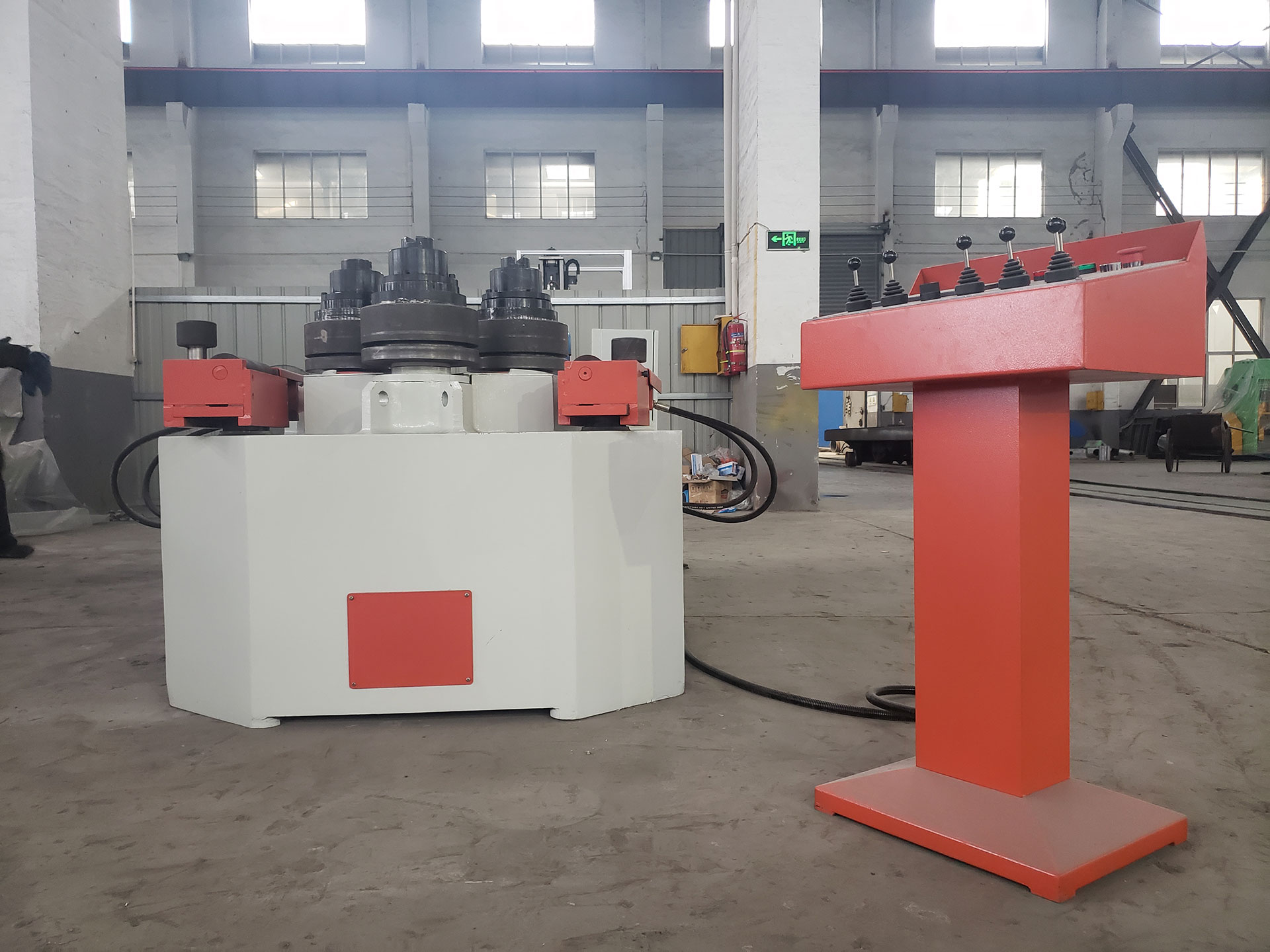# What is the unit of bending stiffness?The unit of bending stiffness, often denoted as “EI,” depends on the units used for the individual components in the formula. The formula for bending stiffness is:

EI=EI

Where:

• EI is the bending stiffness.
• E is the modulus of elasticity of the material.
• I is the second moment of area of the cross-section.

The typical unit of modulus of elasticity (E) is Pascals (Pa) or gigapascals (GPa), depending on the unit system being used.

The unit of the second moment of area (I) depends on the specific shape of the cross-section and the units of measurement used for its dimensions. For common cross-sectional shapes:

• If I is calculated in square meters (m2), then the unit of bending stiffness (EI) will be in Pascals times meters to the fourth power (Pam4).
• If I is calculated in square millimeters (mm2), then the unit of bending stiffness (EI) will be in Pascals times millimeters to the fourth power (4Pamm4).

In practice, engineers often work with consistent units, and the most common unit for bending stiffness is usually N·m^2 (Newton-meters squared) or kN·m^2 (kilonewton-meters squared) in the International System of Units (SI).